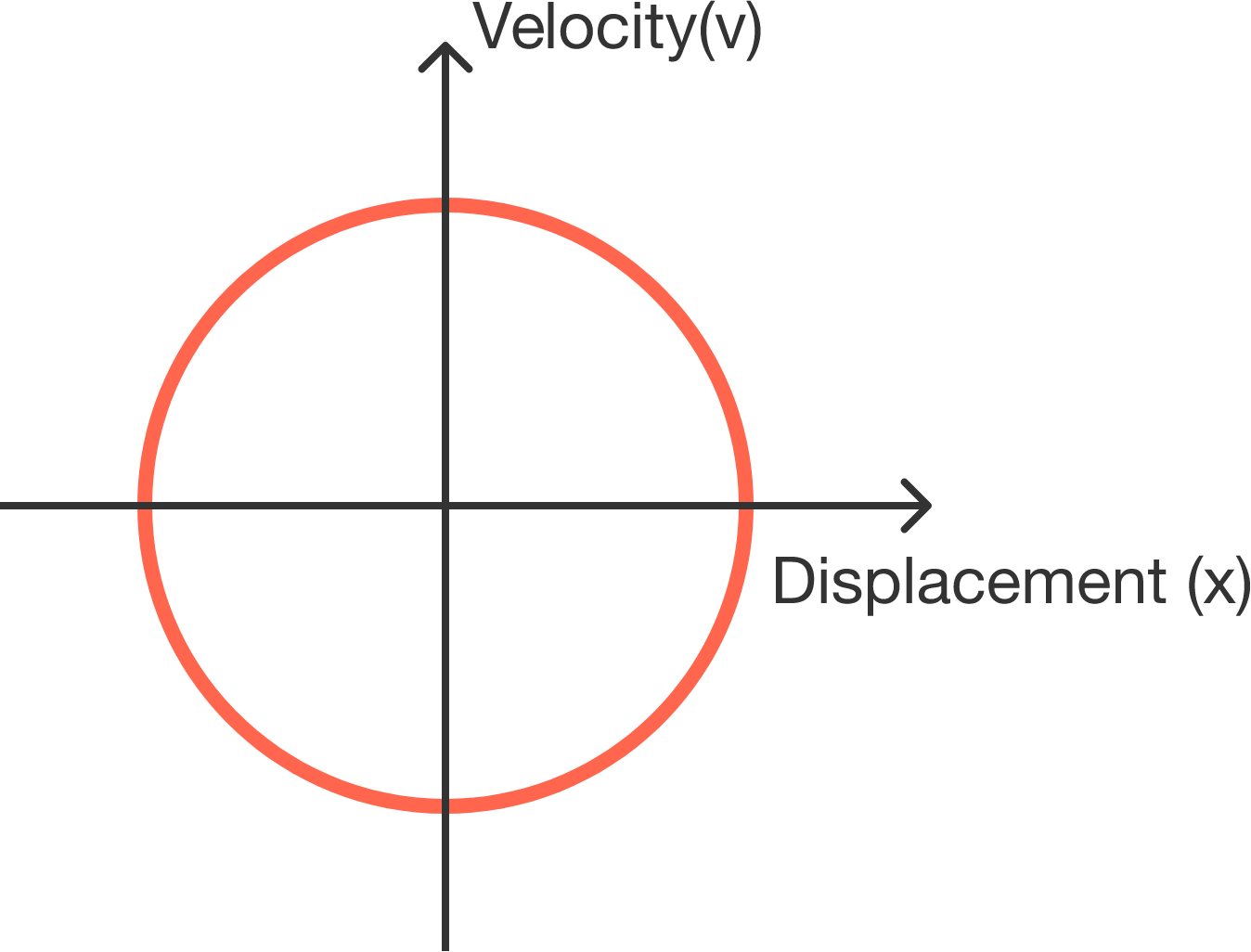# Guess the acceleration from the graphA particle moves in 1-dimension. If we plot its velocity and displacement over time, the trajectory forms a circle that's centered at the origin.

Which of the following relations is true regarding its acceleration $(a),$ velocity $(v),$ and displacement $(x)$?

Note: In the options, $k$ is a positive constant.

×